# Length - examples - page 25

1. Ping time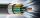Calculate theoretical ping time between Orlando and Shenzhen which is 14102 km distant. Ping time measures the round-trip time for small messages sent from the origin to a destination that is echoed back to the source. The name comes from active sonar term
2. Proportion 2A car is able to travel 210 km in 3 hours. How far can it travel in 5 hours? Put what kind of proportion is this and show your solution.
3. A tileA tile setter is covering 5ft by 5ft square shower wall. Each tile covers 4 5/8in by 4 5/8in square. How many rows of tile are needed to reach 5ft? How many tiles are needed to cover 5ft by 5ft square
4. The positionThe position of a body at any time T is given by the displacement function S=t3-2t2-4t-8. Find its acceleration at each instant time when the velocity is zero.
5. Walking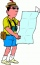Lucie can walk about 3 4/5 miles each hour. How far can she walk in 2 hours 45 minutes?
6. Flooring a roomFind the cost of flooring a room 6.5 m by 5 m with square tiles of sides 25 cm at the rate of rupees 9.40 per tile.
7. Bob traveled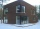Bob traveled 20 meters to Dave's house. He arrived in 3 minutes. What was his average speed for the trip.
8. After 2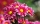After we planted flowers in 2/5 of our garden, 24m remained unplanted. How many meters is the garden in total? If the total area of the garden is 1, the proportion of the remaining area is?
9. Feet to milesA student runs 2640 feet. If the student runs an additional 7920 feet, how many total miles does the student run?
10. Wood dividingWhich equation calculates the number of 1/3-foot pieces that can be cut from a piece of wood that is 7 feet long?
11. Jose and Kaitlyn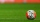Jose and Kaitlyn have a contest to see who can throw a baseball the farthest. Kaitlyn wins, with a throw of 200 ft. If Jose threw the ball 3/4 as far as Kaitlyn, how far did Jose throw the ball?
12. Two boatsTwo boats are located from a height of 150m above the surface of the lake at depth angles of 57° and 39°. Read the distance of both boats if the sighting device and both ships are in a plane perpendicular to the surface of the lake.

We apologize, but in this category are not a lot of examples.
Do you have an interesting mathematical example that you can't solve it? Enter it, and we can try to solve it.

To this e-mail address, we will reply solution; solved examples are also published here. Please enter e-mail correctly and check whether you don't have a full mailbox.

Do you want to convert length units?